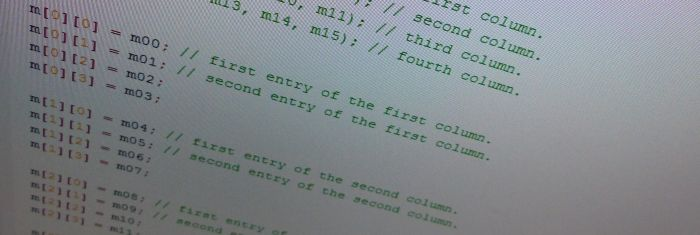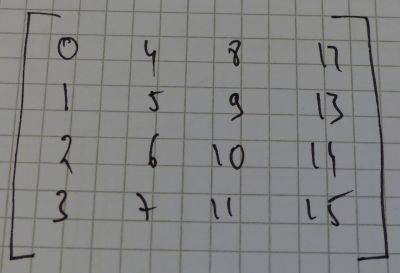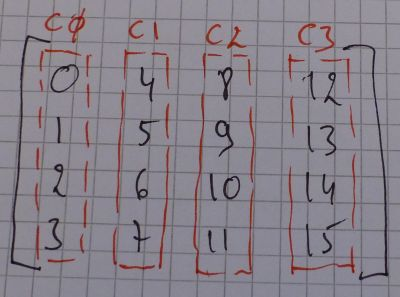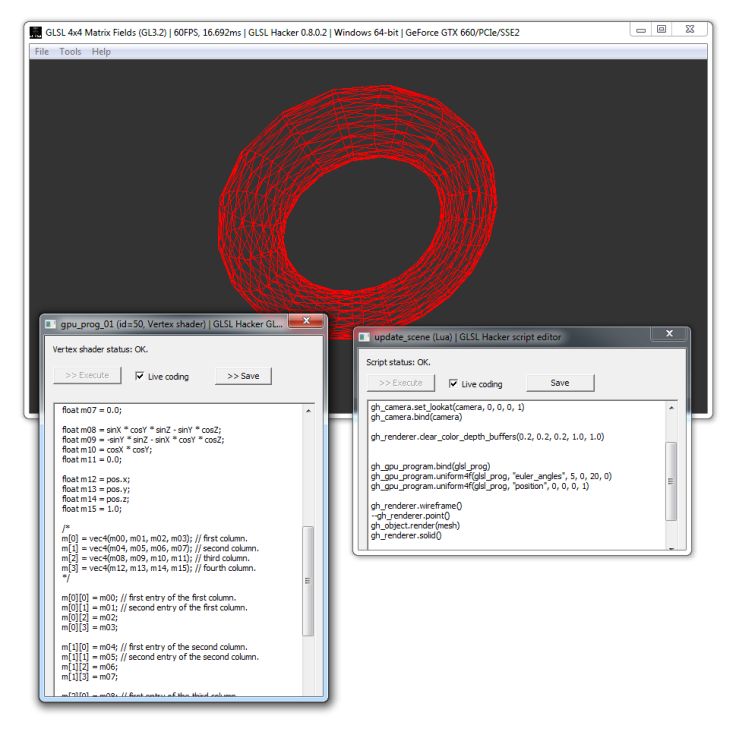# GLSL 4×4 Matrix FieldsHere is a short article about manipulating GLSL mat4 fields. In C, an OpenGL 4×4 matrix is a 16-float array:

```float c_matrix;
```

In GLSL the same matrix is:

```mat4 glsl_matrix;
```

Let’s see how to map the C matrix to the GLSL one and vice-versa. Matrices in OpenGL are column-major. The c_matrix[] can be represented by:The first column is made up of entries 0, 1, 2 and 3. The second column is 4, 5, 6, 7 and so on.

In C, the first entry of the first column is:

```c_matrix;
```

While the second entry of the third column is:

```c_matrix;
```

Now in GLSL. The first entry of the first column is:

```glsl_matrix;
```

The second entry of the third column is:

```glsl_matrix;
```

A mat4 can be seen as four vec4 vectors:

```vec4 c0 = glsl_matrix.xyzw;
vec4 c1 = glsl_matrix.xyzw;
vec4 c2 = glsl_matrix.xyzw;
vec4 c3 = glsl_matrix.xyzw;
```The first entry of the first column is now:

```glsl_matrix.x;
```

And the second entry of the third column is:

```glsl_matrix.y;
```

In the case of an object transformation matrix, the 4×4 matrix usually contains the orientation and the position of the object. The orientation is stored in the upper 3×3 matrix, or in terms of a mat4, in the three first entries of the three first columns. The position is stored in the three first entries of the fourth and last column.

Here is now a GLSL code snippet that build a 4×4 transformation matrix from a 3D position and the three Euler’s angles:

```mat4 build_transform(vec3 pos, vec3 ang)
{
float cosX = cos(ang.x);
float sinX = sin(ang.x);
float cosY = cos(ang.y);
float sinY = sin(ang.y);
float cosZ = cos(ang.z);
float sinZ = sin(ang.z);

mat4 m;

float m00 = cosY * cosZ + sinX * sinY * sinZ;
float m01 = cosY * sinZ - sinX * sinY * cosZ;
float m02 = cosX * sinY;
float m03 = 0.0;

float m04 = -cosX * sinZ;
float m05 = cosX * cosZ;
float m06 = sinX;
float m07 = 0.0;

float m08 = sinX * cosY * sinZ - sinY * cosZ;
float m09 = -sinY * sinZ - sinX * cosY * cosZ;
float m10 = cosX * cosY;
float m11 = 0.0;

float m12 = pos.x;
float m13 = pos.y;
float m14 = pos.z;
float m15 = 1.0;

/*
//------ Orientation ---------------------------------
m = vec4(m00, m01, m02, m03); // first column.
m = vec4(m04, m05, m06, m07); // second column.
m = vec4(m08, m09, m10, m11); // third column.

//------ Position ------------------------------------
m = vec4(m12, m13, m14, m15); // fourth column.
*/

//------ Orientation ---------------------------------
m = m00; // first entry of the first column.
m = m01; // second entry of the first column.
m = m02;
m = m03;

m = m04; // first entry of the second column.
m = m05; // second entry of the second column.
m = m06;
m = m07;

m = m08; // first entry of the third column.
m = m09; // second entry of the third column.
m = m10;
m = m11;

//------ Position ------------------------------------
m = m12; // first entry of the fourth column.
m = m13; // second entry of the fourth column.
m = m14;
m = m15;

return m;
}
```

Remark: manipulating mat4 fields can be dangerous. For example this code does not generate errors nor warnings (at least with my GTX 660 on Windows) but does not work at all:

```  //------ Position ------------------------------------
m = m12; // first entry of the fourth column.
m = m13; // second entry of the fourth column.
m = m14; // ERROR!
m = m15;
```

In the third line, we set m14 to an out of range memory location. Such kind of bugs can be hard to detect…

If you wish to quick test mat4 fields, I prepared a little GLSL Hacker demo you can find in the code sample pack (host_api/GLSL_Misc/mat4_transform/demo.xml).Some references

## 3 thoughts on “GLSL 4×4 Matrix Fields”

1.Damian Trebilco

I will have to double check but I believe your memory layout ordering is wrong.

OpenGL is column major, but is laid out in memory in column order.

Eg. vec4 columns; // First column is the first 4 memory slots

A little known fact is that the memory layout of OpenGL matrices is the same as DirectX!
(eg glTranslatef and D3DXMatrixTranslation should return the same in memory results)
http://www.mindcontrol.org/~hplus/graphics/matrix-layout.html

2.slimy

openGL is actually row major. People are confused by it because when they are looking at the modelview matrix they are looking at model * view and don’t consider that view is a transposed matrix.

Strictly model matrices (ie what you would pass to glMultMatrix) are row major. The first 4 values are the right vector. The next 4 the up vector, the next 4 the forward vector and the last 4 translation.

3.oldcrow

This whole row-major vs column-major thing is really confusing because it depends how you look at your matrices; some books place the homogeneous components in the last column and use pre-multiplication in order to transform vectors, while other books place the homogeneous components in the last row of the matrix and use post-multiplication. Depending on how you are accustomed to writing your matrices, you may think that memory layout in OpenGL is row-major or column-major. The easiest way to avoid confusion is to drop the whole “row- column-major” thing and just remember that OpenGL places the translation components of matrices at the 13th, 14th, and 15th elements of a 16-element array. And as Damian Trebilco pointed out, both Direct3D and OpenGL use the same matrix layout.